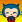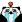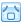# Linux最常用的20条命令

2.3 .1符号微积分

求极限

limit(f,x,a) 求f(x)中x趋近于a的极限值

``>> clear >> syms k x >> lim_t=limit((1-1/x)^(k*x),x,inf)   lim_t =   1/exp(k)``

diff(f,x,n) 求f(x)的n阶导数

``>> clear >> syms x >> dfdx=diff(x^3,x,1)   dfdx =   3*x^2``

jacobian(f,v)  求多元向量函数 f(v) 的雅克比矩阵（高级）

taylor(f,n,x,a) 把f（x） 在x=a 处展开为幂级数 （泰勒级数）

2.3.2 序列/级数的符号求和

s=symsum(f,x,a,b) 求f(x) 从a到b的和

x缺省时findsym自动确认，a,b可同时缺省 默认为 [0,x-1]

f是矩阵时，求和将对元素逐个进行

``>> clear >> syms t k >> f=[t,k^3]; >> s=simple(symsum(f))   s =   [ t^2/2 - t/2, k^3*t]``

2.3.3 符号积分

int(f,x) 求f(x)dx 的不定积分

int(f,x,a,b) 从a到b的定积分

``>> clear >> syms x >> f=sqrt((x+1)/x)/x; >> s=int(f,x),s=simple(s)   s =   - 2*(1/x + 1)^(1/2) - 2*atan((1/x + 1)^(1/2)*i)*i     s =   - 2*(1/x + 1)^(1/2) - 2*atan((1/x + 1)^(1/2)*i)*i``

。。结果怎么整成复数了。。

``>> clear >> syms a b x >> f=[a*x,b*x^2;1/x,sin(x)]; >> disp('the ans is'); the ans is >> int(f)   ans =   [ (a*x^2)/2, (b*x^3)/3] [    log(x),   -cos(x)]   >> pretty(int(f))     +-                 -+    |      2        3   |    |   a x      b x    |    |   ----,    ----   |    |    2        3     |    |                   |    |  log(x), -cos(x)  |    +-                 -+``

``>> clear >> syms x y >> int(int(x*y,y,2,3),x,1,2)   ans =   15/4``

## 20条评论COD试剂 says: 回复[抽耳光][电影] 新手报到，认真学习